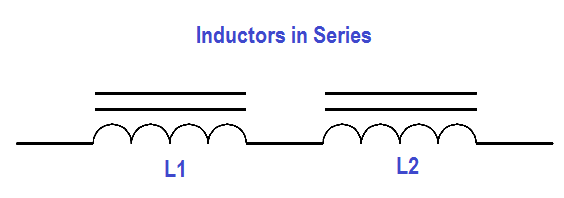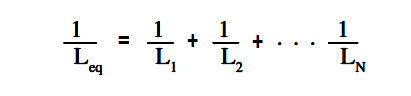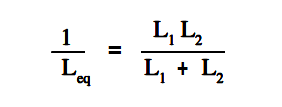# Series and Parallel Inductors

#### Inductors in Series

Inductors in series are combined like resistors in series as shown in below figure.Figure 4 : Inductors in Series

Equivalent inductance (Leq ) of two inductors in series (Figure 4) is given by below Equation.

#### Inductors in parallel

Inductors in parallel are combined like resistors in parallel as shown in below figure.Figure 5 : Inductors in Parallel

The equation for parallel inductors isWhen only two inductors are in parallel, as shown in Figure 5, The above Equation may be simplified as given in below Equation.The above Equation is valid when there are only two inductors in parallel.

1.